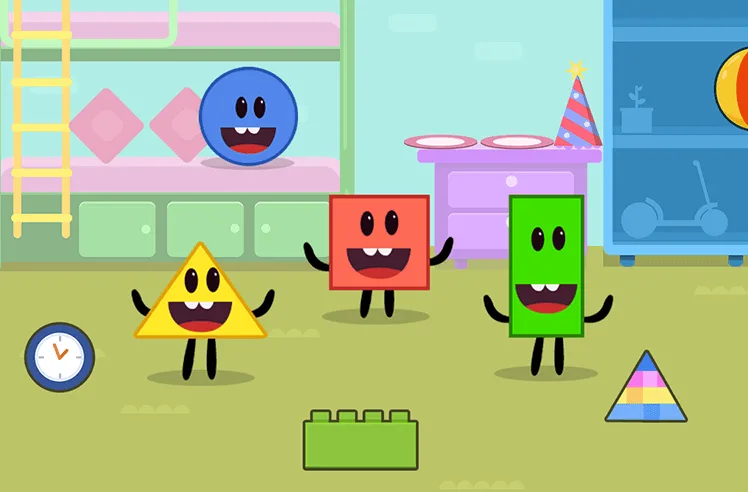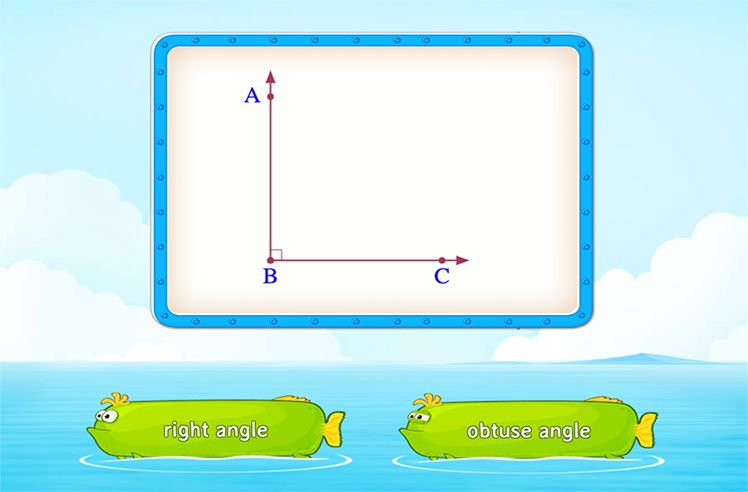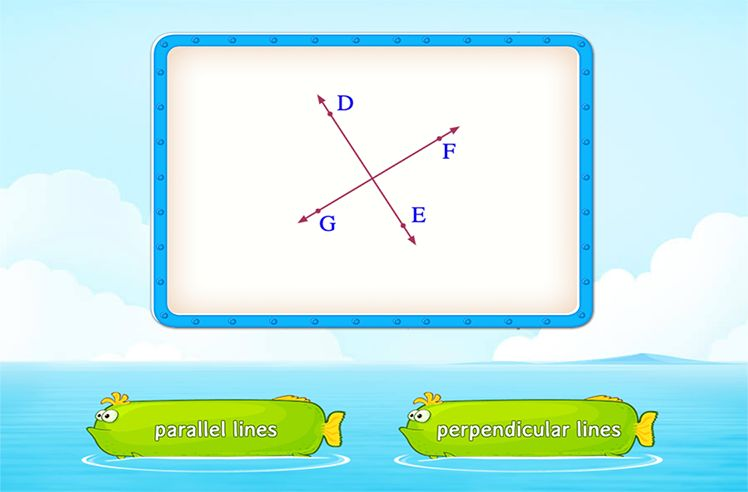# Cone – Definition with Examples

» Cone – Definition with Examples

## What is Cone?

A cone is a distinctive three-dimensional geometric figure that has a flat surface and a curved surface, pointed towards the top. The pointed end of the cone is called the apex, whereas the flat surface is called the base. This is what a cone looks like:

The three main properties of a cone are as follows:

• It has one circular face.
• It has zero edges.
• It has one vertex (corner).

Types of Cones:

A cone can be of two categories, depending upon the position of the vertex on the base:

• right circular cone is one whose apex is perpendicular to the base, which means that the perpendicular line falls exactly on the center of the circular base of the cone. In the image below, h represents the height of the cone, and r  is the radius.
• If the position of the vertex is anywhere apart from the center of the base, then it is an oblique cone

Cones Around Us:

Cones can be found in a variety of things we see everyday. Some examples are mentioned below:

• A funnel is shaped like a cone.
• An ice cream is scooped in a conical pastry.
• The barriers we see on roads are also conical.
• The birthday hats are conical in shape.Shapes

Play NowAngles

Play NowParallel and Perpendicular Lines

Play Now